# How to Use VLOOKUP in Excel Online

## How to Use VLOOKUP in Excel Online

When you’re staring at endless rows of data in an Excel spreadsheet, it’s easy for all that information to turn into one blurry mess. Then there’s the matter of extracting specific data. In addition to spending what feels like an eternity scrolling through the spreadsheet to find what you need, you then second-guess if you actually pinpointed the right data.

That’s where VLOOKUP in Excel comes in: it takes the guesswork out of finding and retrieving data in spreadsheets.

Here, I’ll show you how to use VLOOKUP in Excel, plus give you some tips to make the function even more powerful.

Here’s what we’ll cover:

Note: I use the web version of Microsoft Excel, but the steps are the same in the desktop app.

## What is VLOOKUP in Excel?

The VLOOKUP function in Excel searches for a value in one column based on a given value in another column. The formula is made of four parameters (or arguments):

• Lookup value: this is the value you want Excel to search for.

• Table array: this is the cell range containing the lookup value and the value you want Excel to return (the data you’re looking for). Note: The lookup value must be in the first column in the given range. For example, if your lookup value is in cell `A3`, then your range should start with `A`

• Column index number: this is the column number in the given range containing the value you want Excel to return. If your table array is `A2:D10`, for example, count column `A` as your first column, column `B` as your second, and so on. If your table array is `C2:F10`, count column `C` as your first column, column `D` as your second, and so on. Your column index number tells Excel which column to retrieve the data you’re looking for.

• Range lookup: this is an optional parameter. By default, the VLOOKUP function always returns an approximate match (designated by `TRUE`). If you want an exact match, enter `FALSE`.

Put those parameters together and you get this:

`=VLOOKUP(lookup value,table array,column index number,range lookup)`

## How to use VLOOKUP in Excel

If you’re looking for a quick refresher, here’s the short version of how to use the VLOOKUP formula in Excel. (Keep scrolling for a more detailed breakdown.)

1. Click the cell where you want Excel to return the data you’re looking for.

2. Enter `=VLOOKUP(lookup value,table array,column index number,range lookup)`.

3. Press Enter or Return.

Now let’s dive into a detailed breakdown of how to use VLOOKUP (or vertical lookup).

To keep this tutorial simple, I’ll show you how to use the VLOOKUP function in Excel to identify an employee’s ID based on their last name. Specifically, we’re looking for Sandra Kwon’s employee ID. While you’d probably use VLOOKUP for something more complex with a much larger dataset, the steps to use VLOOKUP remain the same.

A quick reminder before we get started: the lookup value must be in the first column of your table array. For this demo, our lookup value (Kwon in cell `B6`) will be in the first column of our table array (`B2:D10`). If you’re working with a different dataset where the lookup value isn’t in the first column, you may have to reorganize your data. Or you can copy and paste the columns you’re working with into another area of your worksheet. If you go with the latter, I recommend pasting the data into a new worksheet altogether to keep your data manageable.

As a refresher, we want to know Sandra Kwon’s employee ID based on her last name.

1. Click the cell where you want Excel to return the data you’re looking for. In this case, click cell B13.

2. Enter `=VLOOKUP`.

3. Press Enter or Return. Excel will automatically add a left parenthesis after the function, so it looks like this: `=VLOOKUP(`

4. Input the following parameters immediately after the parenthesis, separating each one with a comma.

• Lookup value: `B6`

• Table array: `B2:D10`.

• Column index number: `3`. Remember: the value we want Excel to return (employee ID) is in column D, which is the third column of the given cell range.

• Range lookup: enter `FALSE` to get an exact match.

5. Enter the right parenthesis (`)`) to close your formula so that cell B13 now reads `=VLOOKUP(B6,B2:D10,3,FALSE)`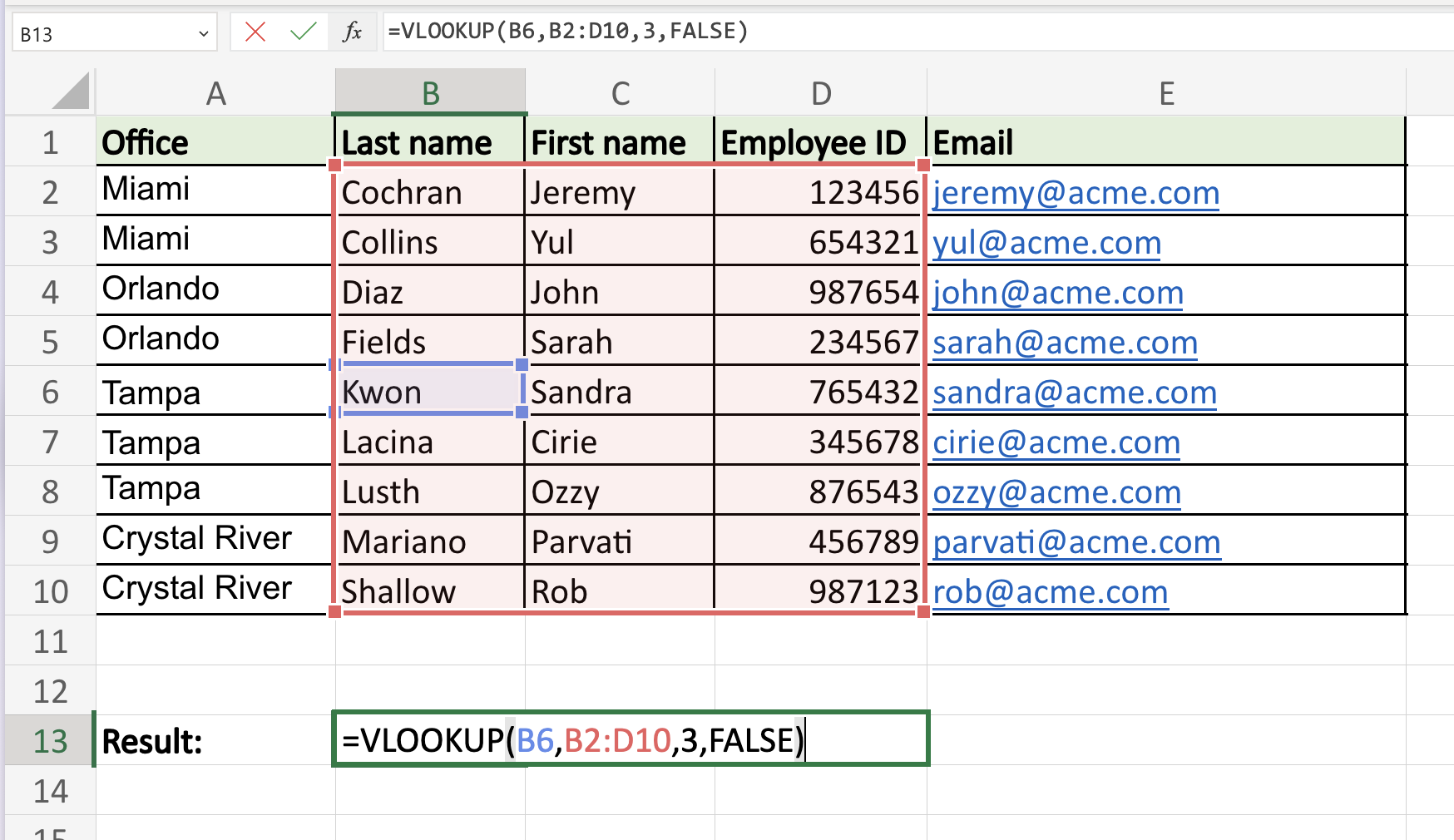6. Press Enter or Return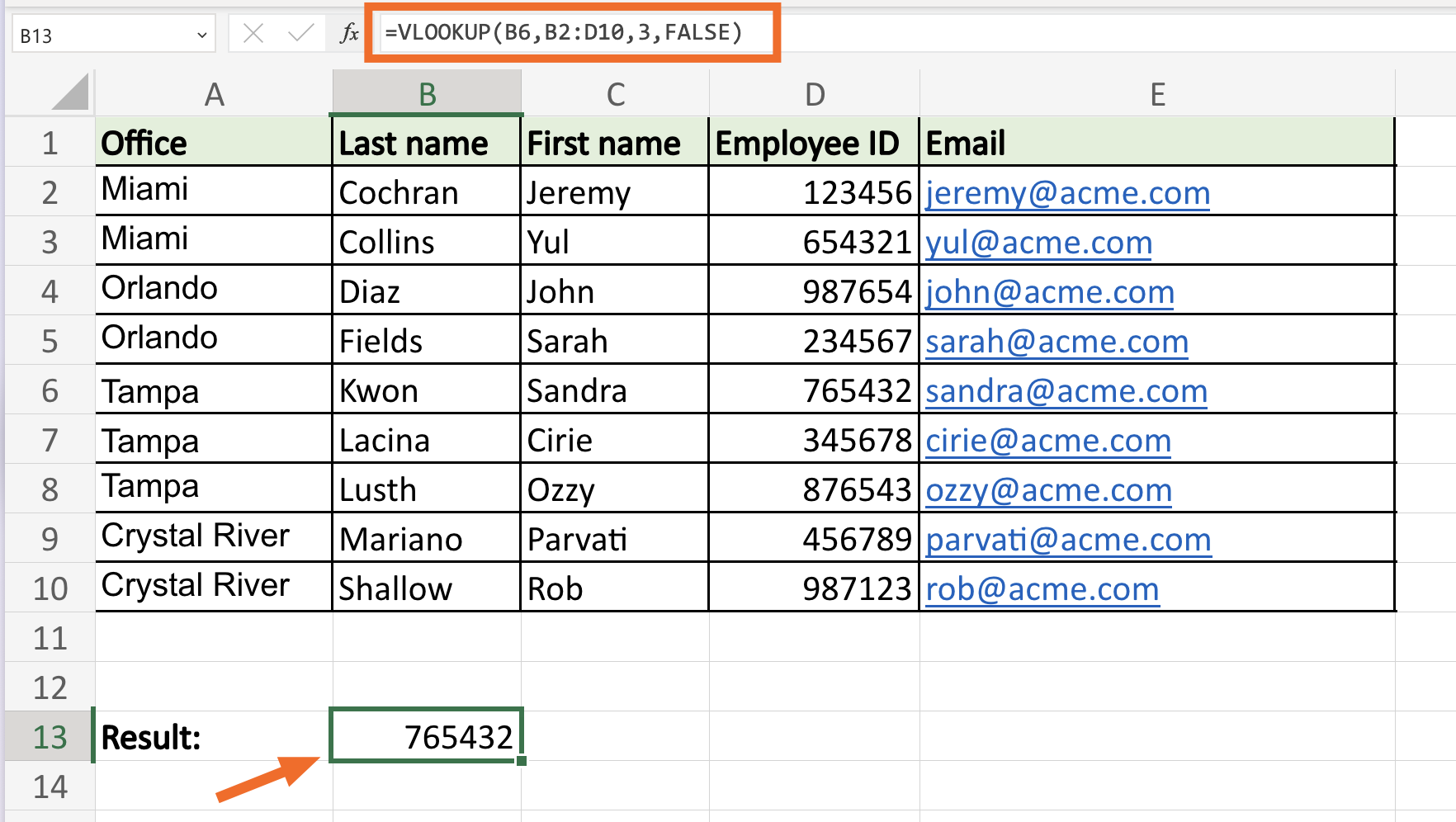Excel immediately returns the corresponding value: `765432`.

## How to do VLOOKUP in Excel with two spreadsheets

Let’s say Sheet 1 of our demo workbook is our primary spreadsheet—it contains every bit of employee data. There’s also a second spreadsheet (Sheet 2), which contains only employee names and their updated company email addresses.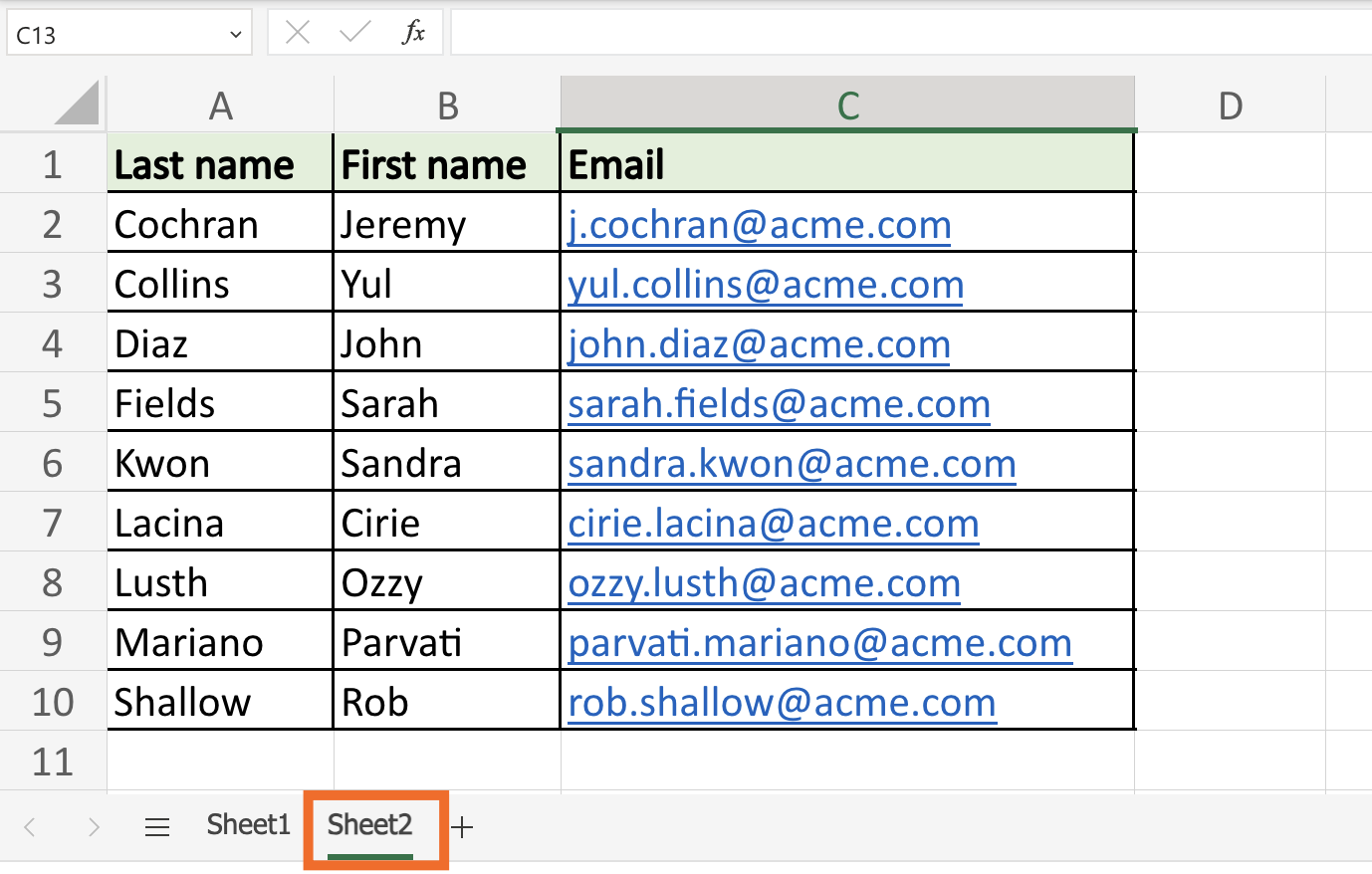Now you need to update the email addresses in Sheet 1 with the new email addresses from Sheet 2. You can accomplish this with the VLOOKUP function, but you’ll need to modify your table array parameter to tell Excel which spreadsheet contains the corresponding lookup value you want it to return.

This is the modified VLOOKUP formula to return a value from another sheet within the same workbook:

`=VLOOKUP(lookup value,sheet!range,column index number,range lookup)`

Let’s use VLOOKUP to update the email address in cell `E2` of Sheet 1 with the email address in cell `C2` of Sheet 2

1. Click cell E2 of Sheet 1.

2. Enter `=VLOOKUP(B2,Sheet2!\$A\$2:\$C\$10,3,FALSE)`. Here’s a breakdown of the modified table array:

• Sheet2!: This is the name of the spreadsheet that contains the given cell range. Note: to reference another worksheet, input `[name of sheet]!`. If your sheet name contains spaces or non-alphabetical characters, it must be enclosed in single quotation marks. For example, `'Sheet 1'!`.

• \$A\$2:\$C:\$10: The cell range is A2:C10. To prevent the range from changing when copying the formula to other cells, we lock it in using absolute cell references

3. Press Enter or Return.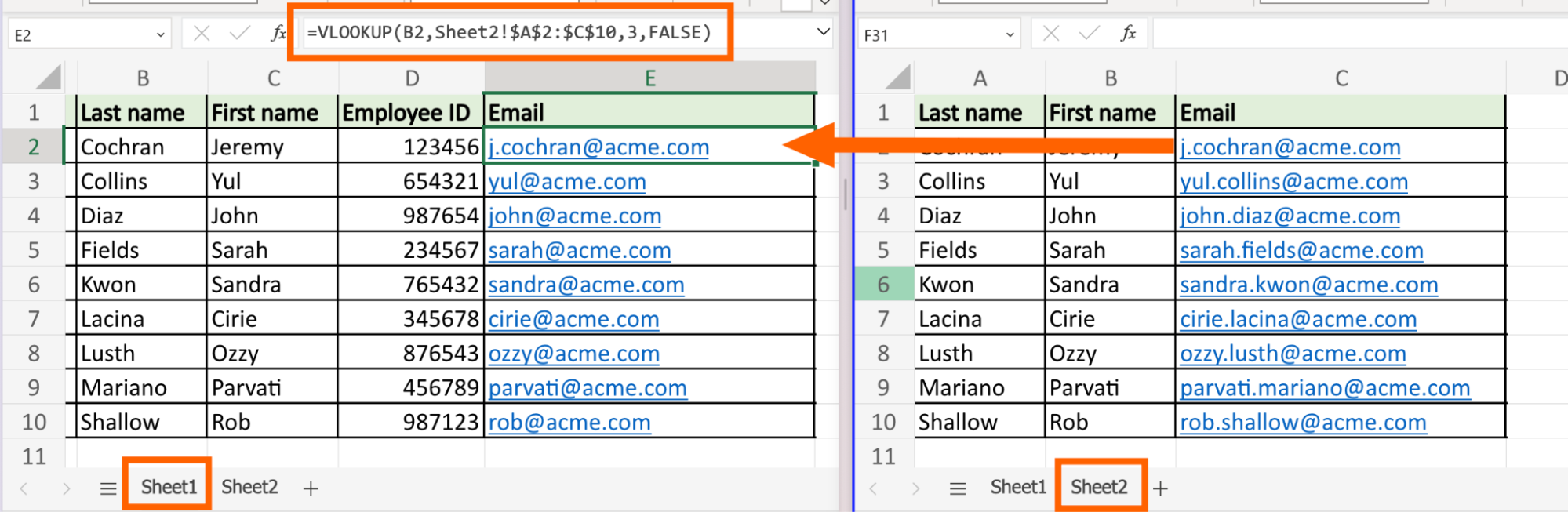Excel returns the corresponding value from Sheet 2 in cell `E2` of Sheet 1: `j.cochran@acme.com`

To quickly update the remaining email addresses in Sheet 1, drag the fill handle from cell `E2` down.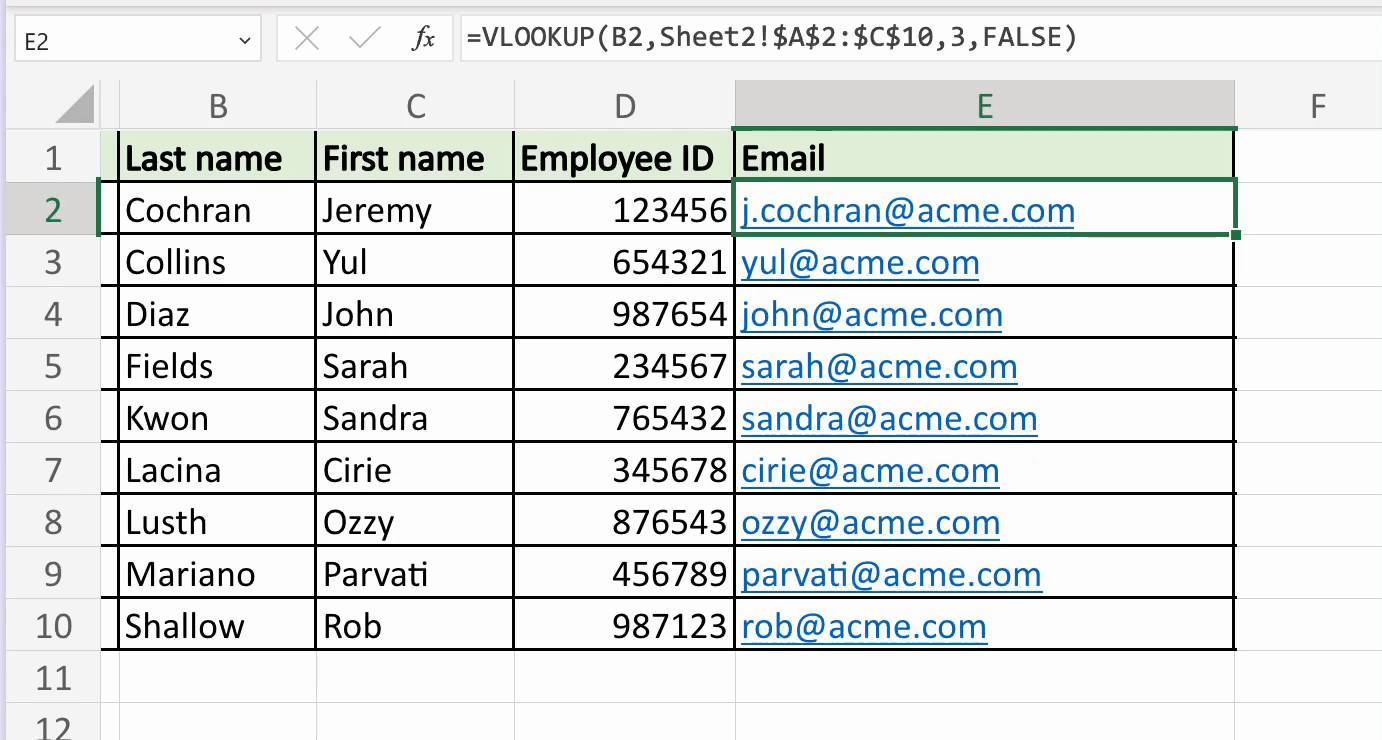## How to do VLOOKUP in Excel with two workbooks

To use VLOOKUP to retrieve data from another workbook, all you have to do is include the file name of the other workbook within square brackets immediately followed by the sheet name and table array. Here’s the formula template:

`=VLOOKUP(lookup value,[file_name.xlsx]Sheet!range,column index number,range lookup)`

Let’s say we stored the employees’ updated email addresses in Sheet 1 of the `2023_employee_emails.xlsx` workbook instead. To populate the new email address in cell E2 of our primary spreadsheet, enter:

`=VLOOKUP(B2,[2023_employee_emails.xlsx]Sheet1!\$A\$2:\$C\$10,3,FALSE)`

## Automate Microsoft Excel

Manual data entry is ripe for human error. With Zapier, you can connect Excel with your go-to apps so you can automate spreadsheet-related tasks. For example, you can automatically add new form submissions to an existing workbook. Learn more about how to automate Excel, or get started with one of these workflows.